nextnano.com  nextnano³  Download | Search | Copyright | Publications  * password protected nextnano³ software2D DG MOSFET (QM)

# nextnano3 - Tutorial

## Ultrathin-body Double Gate FET - DG MOSFET (Double Gate Metal Oxide Semiconductor Field Effect Transistor) (5 nm)

Author: Stefan Birner

If you want to obtain the input files that are used within this tutorial, please check if you can find them in the installation directory.
If you cannot find them, please submit a Support Ticket.
``` -> 2DDoubleGateMOSFET_cl.in  -> 2DDoubleGateMOSFET_qm.in```

## Ultrathin-body Double Gate FET - DG MOSFET (Double Gate Metal Oxide Semiconductor Field Effect Transistor) (5 nm)

Some of these figures are included in this publication:

Modeling of semiconductor nanostructures with nextnano³
S. Birner, S. Hackenbuchner, M. Sabathil, G. Zandler, J.A. Majewski, T. Andlauer, T. Zibold, R. Morschl, A. Trellakis, P. Vogl
Acta Physica Polonica A 110 (2), 111 (2006)

### Structure

The main idea of a Double Gate MOSFET is to control the Si channel very efficiently by choosing the Si channel width to be very small and by applying a gate contact to both sides of the channel. This concept helps to suppress short channel effects and leads to higher currents as compared with a MOSFET having only one gate.

The structure consists of an intrinsic Si channel having the length 25 nm and the width 5 nm.
(The width of 25 nm corresponds to the 65 nm technology node.)
This channel is connected to heavily n-type doped source and drain regions of length 10 nm each (constant doping profile with a concentration of 1 x 1020 cm-3, fully ionized).
The gates have a length of 25 nm and are separated from the Si channel by a 1.5 nm thick oxide layer.
(SiO2,``` static-dielectric-constants = 3.9d0```)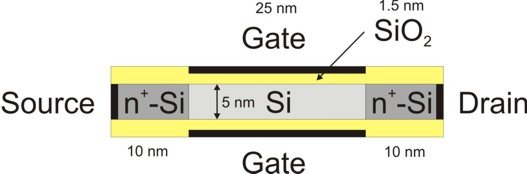The Double Gate MOSFET contains the following regions

 cluster region 1 1 Source contact 2 2 n-type doped source region (Si) 3 3 Si channel (undoped) 4 4 n-type doped drain region (Si) 5 5 Drain contact 6 6 SiO2 7 7 Top gate 7 8 Bottom gate

Both the regions 7 (top gate) and 8 (bottom gate) form the cluster no. 7.

Along the channel direction (x direction), the grid lines are separated by 1 nm (48 grid points),
along the y direction, the grid lines have a 0.5 nm separation (21 grid lines).

The total number of grid points is 48 x 21 = 1008.

### Contacts

We apply a voltage of VD = 0.5 V to the drain contact.
The gate voltage is varied from -0.3 V to 1.0 V in steps of 0.1 V (```=> \$voltage-sweep```).
At the gate a Schottky barrier of 3.075 V is assumed to mimick the gate electrode work function which has been assumed to be 4.1 eV.

``` \$poisson-boundary-conditions   poisson-cluster-number  = 1          ! ```Source```   region-cluster-number   = 1   applied-voltage         = 0.0d0      ! 0.0 [V]   boundary-condition-type = ohmic   contact-control         = voltage   poisson-cluster-number  = 2          ! ```Drain```   region-cluster-number   = 5   applied-voltage         = 0.5d0      ! 0.5 [V]   boundary-condition-type = ohmic   contact-control         = voltage   poisson-cluster-number  = 3          ! ```Gate```   region-cluster-number   = 7   applied-voltage         = -0.3d0     ! -0.3 [V] ... 1.0 [V]   boundary-condition-type = Schottky   schottky-barrier        = 3.075d0    ! phi_B   contact-control         = voltage  \$end_poisson-boundary-conditions  ```

``` \$voltage-sweep   sweep-number            = 1   sweep-active            = yes   poisson-cluster-number  = 3         ! ```Gate:``` poisson-cluster-number = 3   step-size               = 0.1d0     ! ```voltage steps of``` 0.1 [V]   number-of-steps         = 13   data-out-every-nth-step = 1  ```

### Mobility

The mobility is assumed to depend on temperature (T = 300 K) and on the doping concentration but is independent of the electric field.

```  \$simple-drift-models    mobility-model = mobility-model-simba-0  !  => \$mobility-model-simba ```Thus we have two different electron mobilities:

`- `n-type doped Si region: 64.47 cm2/Vs
`  `µ = 55.2 cm2/Vs + 1374.0 cm2/Vs / [ 1 + ( 1.0*1020 / 1.072*1017)0.73  ] = 64.470195 cm2/Vs

`- `intrinsic Si region: 1429.2 cm2/Vs
`  `µ = 55.2 cm2/Vs + 1374.0 cm2/Vs = 1429.2 cm2/Vs

## Results

### Electron density and conduction band profile

The following figure shows a slice through the middle of the device (x=constant), i.e. through the gate contacts.

The source drain voltage is VSD = 0.5 V, and the gate voltage is VG =  0.7 eV.

Two results are shown:

a) classical calculation
self-consistent solution of the two-dimensional Poisson and current equations
The current equation is solved within a drift-diffusion model based on the classical density.

b) quantum mechanical calculation
self-consistent solution of the two-dimensional Poisson, Schrödinger and current equations
The current equation is solved within a drift-diffusion model based on the quantum mechanical density.
The Schrödinger equation has to be solved each time three times because of the different orientations of the
ellipsoidal electron effective mass tensors (mlongitudinal = 0.916 m0 and mtransversal = 0.190 m0).

The Fermi level is almost flat, i.e. constant (-0.249 eV) and very similar in both simulations a) and b).

The conduction band edge in the Si channel is lower in the case of the quantum mechanical simulation b).

The main difference is attributed to the electron density:

a) The classical density has its maximum at the Si/SiO2 interface
because  EF,n `-` EC  has its maximum there, i.e. the conduction
band edge is farthest below the Fermi level.

b) The quantum mechanical density is practically zero at the Si/SiO2 interface because
due to the SiO2 barrier, the wave functions tends to zero at the Si/SiO2 interface.
One can clearly see that the electron density has the highest values in the middle of the channel

and not at the Si/SiO2 interfaces.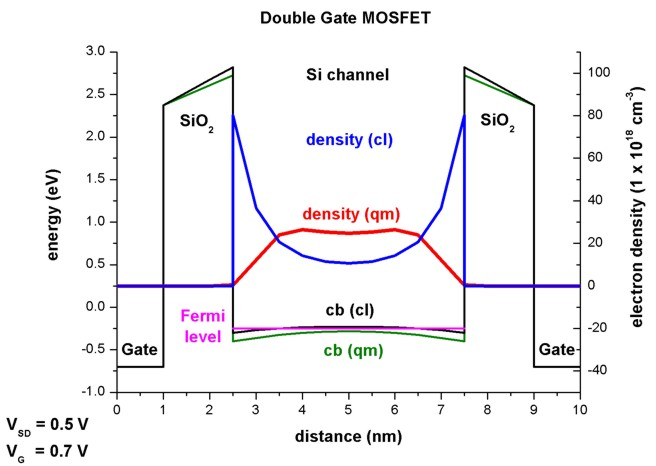The same figures as above but this time
- left figure:   at a gate voltage of 0.3 V (closed channel) and
- right figure: at a gate voltage of 1.0 V (open channel).
The quantum mechanical density has different shapes at different voltages (one maximum in the middle vs. two maxima off-the-center).
(Note: The axes are scaled differently.)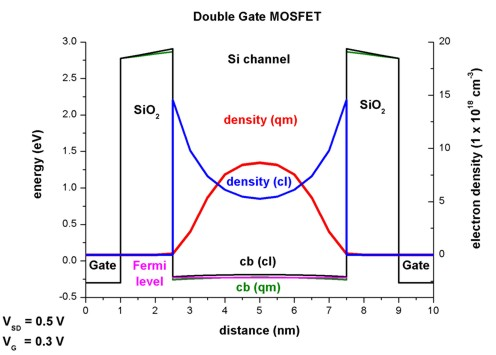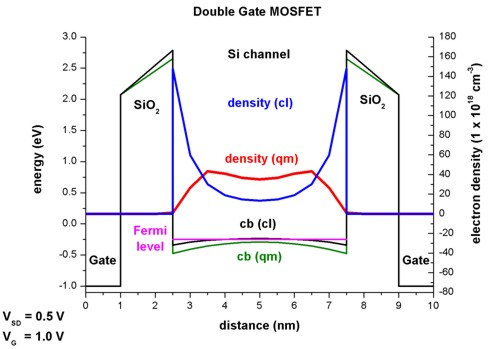### Electron wave functions

There are three Schrödinger equations that have to be solved each time having the following mass tensors that enter into the Hamiltonian H(x,y):

1. mxx = mlongitudinal = 0.916 m0 and myy = mzz = mtransversal = 0.190 m0
2. myy = mlongitudinal = 0.916 m0 and mxx = mzz = mtransversal = 0.190 m0
3. mzz = mlongitudinal = 0.916 m0 and mxx = myy = mtransversal = 0.190 m0

Note that mzz(x,y) does not enter the Hamiltonian but mzz(x,y) is used to calculate the quantum mechanical density (m|| dispersion).
The quantum mechanical density for such a two-dimensional simulation is proportional to the square root of mzz(x,y).

The quantum mechanical density is obtained for each grid point by
- summation over all eigenstates Ei
- evaluation of the square of the wave function psi(x,y)2
- weighting psi(x,y)2 with the Fermi-Dirac integral F-1/2[ (EF - Ei) / kBT ] (which includes the Gamma prefactor of the Fermi-Dirac integral)
- multiplication by a factor (spin and valley degeneracy  / (surface of grid point), square root of (mzz kBT / (2 pi hbar2))

Most of the wave functions are located in the source and drain region (not shown in the figure below).
This figure shows some of the lowest wave functions (psi2) that contribute to the quantum mechancial density in the region where the 1D slice was taken (i.e. in the middle of the device (VG = 0.7 V, VSD = 0.5 V).
The Fermi energy along the 1D slice through the middle of the device lies at -0.249 eV.

The states are labelled from top to bottom:
(Note: They are sorted by energies but their distance is not equivalent to their energy spacing.)

```deg1:    35th state    -0.215 eV ```(psi2 is zero at the 1D slice which can be seen in the right figure)``` deg1:    32nd state    -0.224 eV ```(25 meV above Fermi level)``` deg3:    13th state    -0.226 eV ```(23 meV above Fermi level)``` deg2:    32nd state    -0.250 eV ```(below Fermi level, corresponding to 2nd subband)``` deg2:    25th state    -0.277 eV ```(below Fermi level, corresponding to 1st subband)

`deg1: `These are the states originating from the valleys having the light, transversal mass perpendicular to the channel
(i.e. these states have higher energies).``` deg3: ```These are the states originating from the valleys having the light, transversal mass in the plane of the channel```       ``` mxx = myy = mtransversal = 0.190 m0 (high energies due to light masses)
`deg2: `These are the states originating from the valleys having the heavy, longitudinal mass perpendicular to the channel
as is the case in standard MOSFETs (i.e. these are the states that are occupied because the energies are the lowest).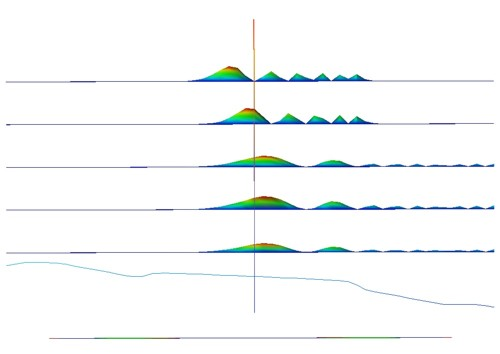### Output

- The band structure (conduction and valence bands) and the electrostatic potential will be saved into the directory` band_structure/`.``` ```- The densities (electron densities) will be saved into` densities/`.``` ```- Current data (Fermi levels, current density, mobility and I-V characteristics) will be saved into` current/`.``` ```- The raw data for the potentials and the Fermi levels (can be read in later in subsequent runs) will be saved into` raw_data/`.

## I-V characteristics (current-voltage characteristics)

• The current-voltage (I-V) characteristic can be found in the following file:` current/IV_characteristics2D.dat`
The drain voltage has been kept constant at 0.5 V, the gate voltage varied from -0.3 V to 1.0 V.

Due to the influence of quantum mechanics a shift of the threshold voltage Vth to a slightly higher voltage can be seen.

```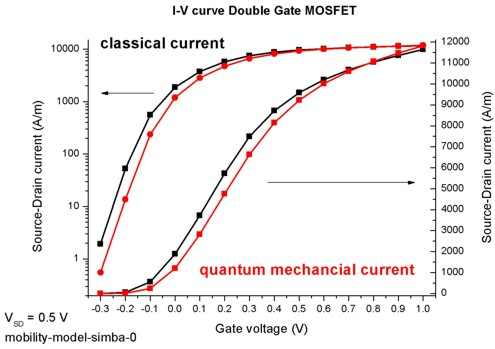```Note that the absolute magnitude of the current is determined mostly by the mobility model.
By using a more realistic mobility model that takes into account the dependency of the parallel and perpendicular electric fields, a smaller current would be obtained.

a) To calculate the I-V characteristics for the classical simulation (13 voltage steps from -0.3 V to 1.0 V), it took about 30 minutes.
(Linux executable for 64-bit Opteron)

b) To calculate the I-V characteristics
for the quantum mechanical simulation (13 voltage steps from -0.3 V to 1.0 V), it took about minutes.
(Windows executable for 64-bit Opteron)